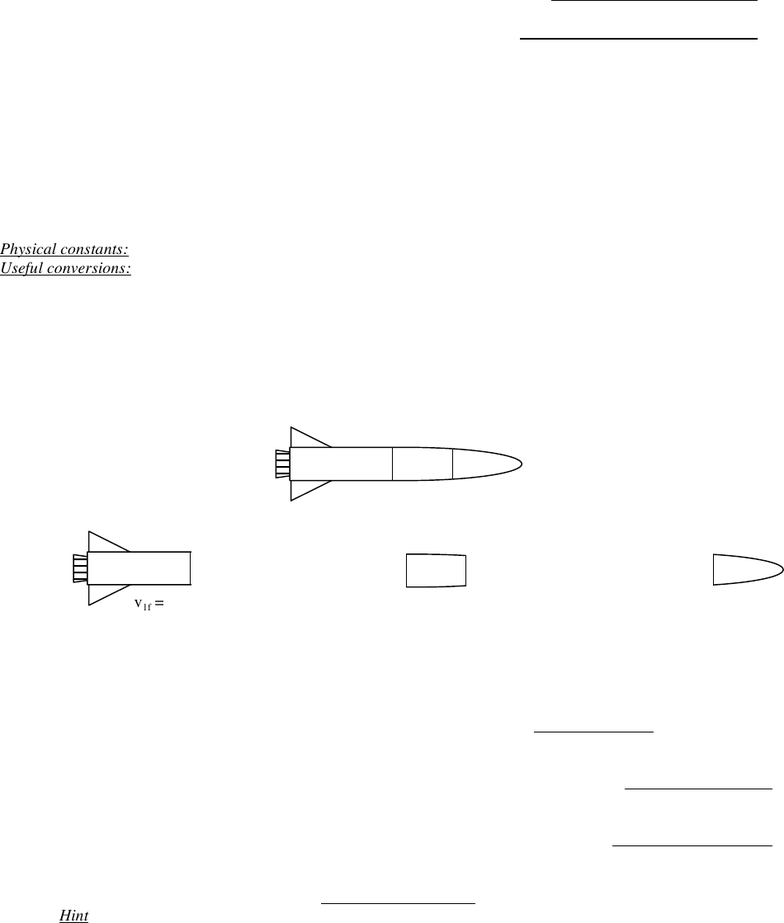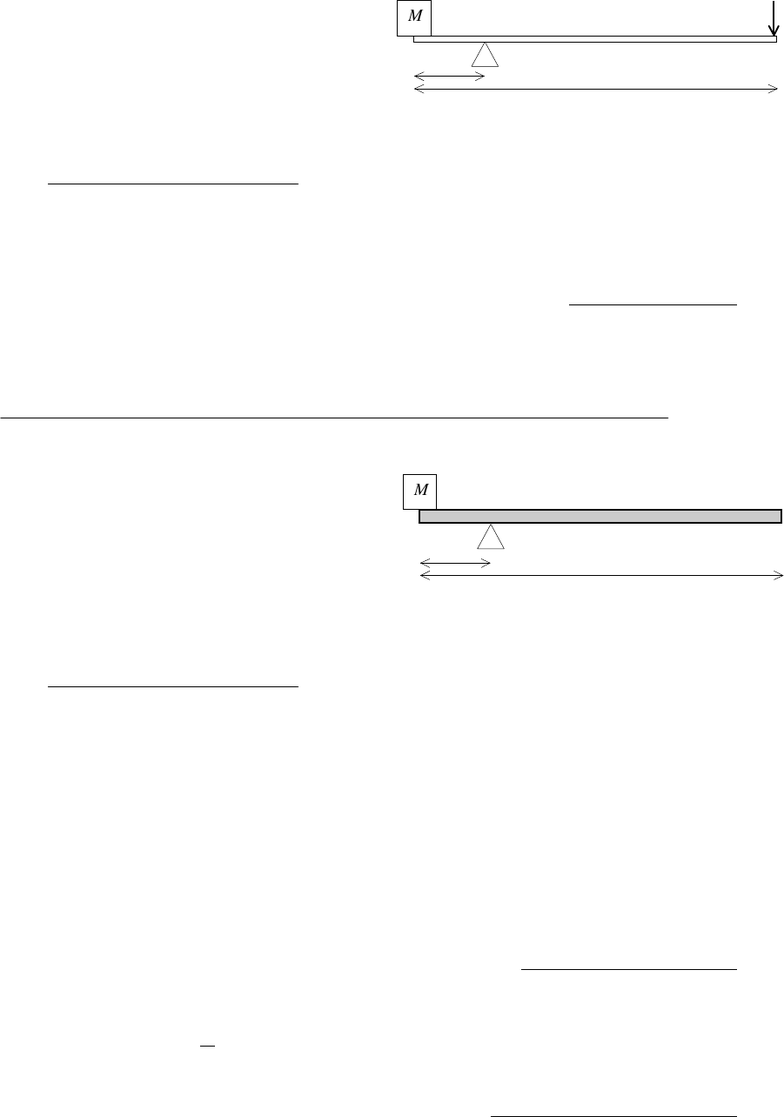Study Guides (400,000)
US (230,000)
UH (60)
PHYS (30)
All (30)
Midterm

# Hawaii PHYS 151 exam151m3-09sprExam

Department
Physics
Course Code
PHYS 151
Professor
All
Study Guide
Midterm

This preview shows pages 1-2. to view the full 6 pages of the document.Physics 151 Roster No.:
Due: Friday, April 24, 2009
Score:
Take-Home Midterm Exam #3, Part A
NO exam time limit. Calculator required. All books and notes are allowed, and you may obtain help
from others. Complete all of Part A AND Part B.
For multiple-choice questions, circle the letter of the one best answer (unless more than one answer is asked
for). For fill-in-the-blank and multiple-choice questions, you do NOT need to show your work.
Show your work on all free-response questions. Be sure to use proper units and significant figures in your
Ignore friction and air resistance in all problems, unless told otherwise.
Physical constants: It’s an open-book test, so you can look them up in your textbook!
Useful conversions: It’s an open-book test, so you can look them up in your textbook!
1. A three-part rocket begins intact as a single object in distant outer space, traveling to the right at 4.0 km/s. The
first “stage” (m1 = 950,000 kg) explodes away from the rear of the rocket, with an unknown final velocity (v1f).
Later, the second stage (m2 = 550,000 kg) explodes away from the rear, with a final velocity to the right at 6.0 km/s.
The third stage (m3 = 350,000 kg) ends up with a final velocity to the right of 13.0 km/s.
(All three masses move only along the x-axis. Ignore gravity throughout this problem. Assume that all
parts of the rocket have constant masses.) For ALL answers to this problem, use positive values for “to the right,”
and negative values for “to the left”:
a. (3 pts.) What is the final velocity v1f of the first stage? ____________________
b. (2 pts.) Find the impulse received by the first rocket stage (in its explosion):
c. (2 pts.) Find the total impulse received by the second rocket stage (after both explosions):
d. (2 pts.) Find the total impulse received by the third rocket stage (after both explosions):
e. (1 pt.) Find the sum of these three impulses:
Hint: According to conservation of momentum, what should the sum of all impulses be equal to?
f. (1 pt.) During the second explosion, the force acting on m2 was _______________ the force acting on m3.
A. less than B. the same as C. greater than
g. (1 pt.) After both explosions, the total kinetic energy of the system
A. increased B. decreased C. remained unchanged
h. (1 pt.) After both explosions, what is the final velocity of the center-of-mass of the three parts? ____________
m2
m1
m3
v1f = ?
m1
m3
m2
v2f = +6.0 km/s
v3f = +13.0 km/s
vi = +4.0 km/s

Only pages 1-2 are available for preview. Some parts have been intentionally blurred.2. A simple lever gives the user a mechanical advantage:
pushing down with a small force Fin on the long end of the
lever creates a large upward force Fout on the short end.
Suppose that you want to support mass M at rest by
using a lever of total length L whose fulcrum is located at a
distance d from the short end of the lever. (Assume that the
mass of the lever itself is negligibly small.)
a. (2 pts.) What is the mechanical advantage (Fout ÷ Fin) of
this lever? Express your answer ONLY in terms of L, d, and
mathematical constants:
b. (1 pt.) At what distance d should the fulcrum be positioned so that the lever has a mechanical advantage equal to
exactly 1? Express your answer ONLY in terms of L and mathematical constants:
c. (1 pt.) As d 0, what value does the mechanical advantage approach?
A. 0 B. 1 C.
We can eliminate the need for a human Fin by instead using a
thick, massive lever with mass m, so that the weight of the
lever itself balances M. (Assume that the lever has uniform
thickness and density.)
d. (2 pts.) At what distance d should the fulcrum be
positioned so that the lever’s mass m exactly balances M?
mathematical constants:
e. (2 pts.; –1 for each error) Which of the following statements is/are TRUE for a balanced system? Circle ALL
that apply:
A. If M >> m, d 0.
B. If M = m, d = L/2.
C. If M << m, d L.
f. (3 pts.) Suppose that d is positioned incorrectly, so that the lever system is NOT balanced. Let d = 25 cm,
L = 100. cm, M = 7.0 kg, and m = 5.0 kg. Immediately after the system is released from horizontal rest
(as shown in the diagram above), what is the net torque about the fulcrum?
g. (3 pts.) In part (f), what is the system’s angular acceleration immediately after it is released? (Assume that
the lever is a thin rod with I =
7
48
mL2, and that M is a “point mass” located at the very end of the lever.)
h. (1 pt.) The angular acceleration in part (g) is
A. clockwise B. counter-clockwise
M
L
d
M
L
d